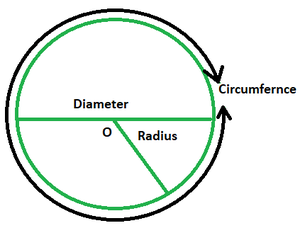# Surface Area of Circle Formula

• Last Updated : 14 Mar, 2022

A circle is made up of points that are all at the same distance from the circle’s centre. A closed geometric form is a circle. In everyday life, we see circles in the form of a wheel, pizzas, a round ground, and so on.Basic Terms

• Radius: The radius of a circle is the distance between its centre and any point on the edge. It is generally denoted as r. A circle has an endless number of radii.
• Diameter: It is a line that runs through the centre and has its ends on the circle. It is denoted as D and is twice the value of the radius of the circle.
• Circumference: The extent of the circle’s border equals its circumference. This signifies that a circle’s perimeter equals its circumference. The circumference of the circle will be equal to the length of the thread that neatly wraps around its perimeter. It is given as 2πr.

### Area of a Circle

The area of a circle relates to the quantity of space encompassed by a circle’s edge. The area filled by the circle is the territory within the circle’s perimeter. It’s also known as the total quantity of square units included within the circle.

Formula

The surface area of a circle is given by the following formula:

A = πr2

where r is the radius of the given circle.

or

A = C2/4π

where C is the circumference of the given circle.

### Sample Problems

Question 1. Find the area of a circle given its radius is 8 m.

Solution:

Given: r = 8 m

Since area of a circle = πr2

A = π(8)2

= 64π

= 200.96 m2

Question 2. Find the area of a circle given its circumference is 12 cm.

Solution:

Given: C = 12 cm

Since, A = C2/4π

= 122/4π

= 11.46 cm2

Question 3. Find the area of a circle given its diameter is 12 cm.

Solution:

Given: D = 12 cm

or, Radius = r = 12/2 = 6 cm

Since A = πr2

= π(6)2

= 113.04 cm2

Question 4. Find the area of a circle given its radius is 9 cm.

Solution:

Given: r = 9 m

Since area of a circle = πr2

A = π(9)2

= 81π

= 254.34 cm2

Question 5. Find the area of a circle given its diameter is 10 cm.

Solution:

Given: D = 10 cm

or, Radius = r = 10/2 = 5 cm

Since A = πr2

= π(5)2

= 78.5 cm2

My Personal Notes arrow_drop_up